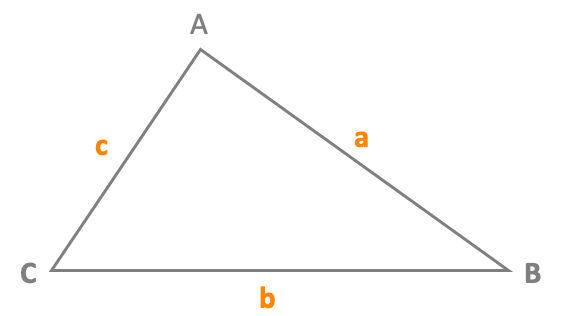# Perimeter of a trianglePerimeter P = a + b + c

AB = a
BC = b
CA = c

## Example

ABC is a triangle with a = 4 cm, b = 3 cm et c = 2 cm
Perimeter P of a triangle ABC = a + b + c = 4 + 3 + 2 = 9 cm

## Calculate the perimeter of a triangle

 Side a = AB Side b = BC Side c = CA Perimeter of the triangle

## Definition of a triangle

A triangle is a polygon having three sides. It is a closed figure with three sides.
A triangle is a three-sided polygon that consists of three edges and three vertices.

To calculate the perimeter of a triangle, add the length of its sides.
The perimeter of a triangle can be calculated by adding the length of all the sides.

The sum of all internal angles of a triangle is always equal to 180°.
A triangle has three sides, three angles, and three vertices.
The sum of any two sides of a triangle is always greater than the other side.

### An isosceles triangle

An isosceles triangle is a triangle having two sides of the same length.
If a triangle is isosceles then its two angles at the base are equal.
If a triangle has two equal angles, then it is isosceles.

### An equilateral triangle

In an equilateral triangle, all three angles are equal and measure 60°.
An equilateral triangle is a triangle whose all three sides are having the same length.

### A right triangle

A right triangle (American English) or right-angled triangle (British) is a triangle in which one angle is a right angle (90°).
A right triangle is a triangle having two perpendicular sides.

## Properties of a triangle

A triangle has three sides and three angles.
The sum of the angles (internal angles) of a triangle is always 180 degrees.
The sum of the length of any two sides of a triangle is greater than the length of the third side.
The sum of all exterior angles of any triangle is equal to 360°.

## To go further

The perimeter of a triangle is the sum of the length of its three sides.